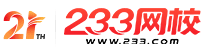#计算机二级

•扫描二维码进入

• 题 库扫描二维码进入

• 移动233网校移动

• 移动APP扫描二维码下载APP

## 2019年3月计算机二级MySQL强化练习及答案(8)

1.若有说明语句：double*p，a;则通过scanf语句正确给输入项读人数据的程序段是( )。

A.*p=&a;scanf("%1f”，p);

B.*p=&a;scanf("%f"，p);

C.p=&a;scanf("%"，*p);

D.p=&a;scanf("%1f"，p);

2.下列程序的输出结果是( )。

#include

main()

{ int a={{1，2，3)，{4，5，6))，(*p)，i;

p=a ;

for(i=0;i<3;i++)

{ if(i<2)

p[i]=p[i]-1;

else

p[i]=1;

}

printf("%d\n"，a[o]+a+a);

}

A.8

B.7

C.12

D.9

3.执行下列程序后，输出的结果是( )。

#include

#define S(X) X*X

void main()

{ int a=9，k=3，m=2;

a/=S(k+m)/S(k+m)：

printf("%d"，a);

}

A.1

B.4

C.9

D.0

4.设x=015，则x=x^017的值是( )。

A.00001111

B.11111101

C.00000010

D.1 1000000

5.若fp是指向某文件的指针，且尚未读到文件末尾，则函数feof(fp)的返回值是( )。

A.EOF

B.-l

C.非零值

D.0

6.设变量a、b、c、d和y都已正确定义并赋值。若有以下if语句：

if(a<B)< p>

if(c==d)y=0;

else y=1;

A.y=0，a<B且C=D< p>

y=1，a≥b

B.y=0，a<B且C=D< p>

y=1，a≥b且c≠d

C.y=0，a<B且C=D< p>

y=1，a<B且C≠D< p>

D.y=0，a<B且C=D< p>

y=1，c≠d

7.下列程序的运行结果是( )。

#include

void main()

{int s=0，k;

for(k=7;k>1;k--)

{switch(k)

{case 1：

case 4：

case 7：

s++：

break;

case 2：

case 3：

case 6：break;

case 0：

case 5：

s+=2：

break;

}}

printf("s=%d"，s);

}

A.s=3

B.s=4

C.s=5

D.无输出结果

8.若i、j已定义成mt型，则下列程序段中内循环体的总执行次数是( )。

for(i=6;i>0;i--)

for(j=0;j<4;j++){…}

A.20

B.24

C.25

D.30

9.下列叙述中正确的是( )。

A.只能在循环体内和switch语句体内使用break语句

B.eotinue语句的作用是结束整个循环的执行

C.在循环体内使用break语句或continue语句的作用相同

D.从多层循环嵌套中退出时，只能使用got0语句

10.有以下程序：

#include

void WriteStr(char*fn.char*str)

{ FILE*fp;

fp=fopen(fn，"w");fputs(str，fp);fclose(fp);}

main()

{ WriteStr("tl.dat"，"start");

WriteStr("t1.dat"，"end");)

A.start

B.end

C.startend

D.endrt

• 海量免费模拟试题，在线测试。

• 各科每年真题试卷，实战演练！

• 逐章逐节针对练习，专项突破。

• 每天10道最新试题，随机练习。

• QQ群号：806931213

扫描二维码加群• ##### 微信订阅号：kszx233

扫描二维码加群